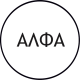Feeds:
Posts

## Only for Geniuses

too early for me!Tease Dat Brain!  What’s the answer: I’ll explain later, so don’t post your reasons, just your answers
Update 4/21: There’s 1 mathematical formula answer so far. The rest are patterns. So far 1 pattern seems to be most popular.
Update 4/17: There are at least 2 correct answers that can be proved. So everyone, if you post a number that hasn’t yet been posted, tell us why as well. Maybe there’ll be more than 2 answers…

Thanks to SMS

### 19 Responses

1. on 2015/05/26 at 15:15 | ReplyDenver

Is one of the answers 90?

2. on 2015/06/10 at 08:42 | Replyjulie

54

3. on 2015/06/11 at 20:20 | ReplyRob

I get 72

4. on 2015/06/11 at 23:15 | ReplyBeck Beck

56

5. on 2015/06/12 at 09:57 | Replyvirginia bermell

6 x 7 42
7 x 8 56
8 x 9 72
9 x 10 90

6. on 2015/06/25 at 15:12 | ReplySridhar

It’s 90

• on 2016/10/04 at 02:07 | ReplyAhmed

Right

7. on 2015/06/26 at 08:46 | Replyfarrahnsmom

9=9
It cannot equal anything else. 1=1, 2=2, 3=3, 4=4 etc

8. on 2015/06/26 at 21:18 | ReplyTeresa Sisson

72

9. on 2015/07/16 at 02:09 | ReplyIsiahM16

Umm dahh… It’s 90. It’s always the original number multiplied by the next number over it

• on 2015/07/16 at 02:11 | ReplyIsiahM16

And the question is “if”… It didn’t say 2=6… It said “if 2=6” so it doesn’t have to be 2=2

10. on 2015/09/04 at 23:59 | ReplySteve

It is obviously 72. each answer is a multiplication of the one before so for example 2=6 means 2 x 3 = 6, 3 being the next number upwards. The reason 9 looks odd is they missed a step BUT if you use the rules the 9=72 – why? Because the missing number is 8 and 8 x 9 = 72. If they showed the whole sequence there would be 8=56 in between 7 and 9 – geddit…..?

11. on 2015/09/07 at 21:22 | ReplyAhmed

72 isn’t correct with that way
1=3

and -1 ect….
9 = any number except 6,12,20,30,42,2,3,4,5,6. This is correct
So for me 9= 13
I think he will start again

12. on 2015/09/22 at 17:29 | Replydani

60
(Add the double of the value to the sum before)

13. on 2015/10/03 at 04:15 | Replyreem

110

14. on 2015/11/06 at 06:18 | ReplyPernille

well i think its 90 cause if you do like this :
2 x 3 = 6
3 x 4 = 7
4 x 5 = 20
5 x 6 = 30
6 x 7 = 42
then its got to be..
9 x 10 = 90

😀 all the numbers got to be multiplied with the number over it 😀

15. on 2016/04/03 at 19:14 | Replyjojo

58

16. on 2016/08/09 at 21:17 | ReplyBecky

Using the pattern provided, 9=81

17. on 2016/10/17 at 08:01 | ReplyShivaram

Difference between 6&2 is 4(2 square), similarly difference between 12&3 is (3 square) and finally difference between 58&9 is(7 square)